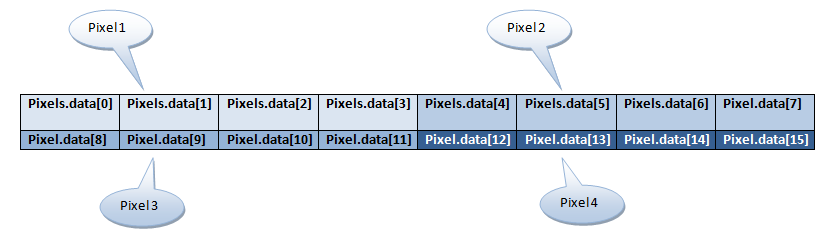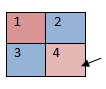Getting image data from canvas

You can read the pixel data on a canvas using the getImageData() method. Image data includes the colour of the pixel (decimal, rgb values) and transparency (alpha value).

The getImageData() allows you to get the pixel data from any rectangular area.

getImageData(x,y,length,breath);

where:
x,y = The starting point of the rectangular area
length = length of the rectangular area
breath = breath of the rectangular area

For ex:

var imageData = ctx.getImageData(10,10,100,50);

The above statement will read the pixels within the rectangule, defined by the starting point (10,10), length 100px and breath 50px. You can also read a single pixel data by setting the length and breath to 1.

var imageData = ctx.getImageData(10,10,1,1);

Return value from getImageData()

You can assign the return value (an ImageData object) of getImageData() to a variable and then draw it back on canvas using the putImageData() method. The colour and transparency information will be stored in an array. If you are reading a single pixel as in

var imageData = ctx.getImageData(10,10,1,1);

You can access the coloru values as follows:

imageData.data - Value of red in decimal (integer between 0 and 255)
imageData.data - value of green in decimal (integer between 0 and 255)
imageData.data - Value of blue in decimal (integer between 0 and 255)
imageData.data - Alpha value (integer between 0 and 255)

If you are reading a bunch of pixels within a rectangle, the length and breath value you provide in the getImageData() method will determine the array size of the return value.

For example, if you have

var Pixel = ctx.getImageData(10,10,2,2);

You are reading the pixel data within a 2 X 2 rectangle. However, the return value, Pixel will be a one-dimentional array of 16 (2 *2 = 4 pixels, with each pixel requiring 4 spaces in the array to store colour and alpha values. So 4 * 4 = 16).Thus, if you want to read the colour information for the fourth pixel,The information will be contained in Pixel.data, Pixel.data, Pixel.data, and Pixel.data.

Finding the size of a pixel object:

The return value from getImageData() will contain both the height and width of the rectangular area from where the colour data was read. To access the width and height, use pixel.width and pixel.height respectively

Notes:

1. If the canvas has images loaded from the hard disk and you try to access pixel information using the getImageData() method, it will not work and will show a security violation "NS_ERROR_DOM_SECURITY_ERR". This is because DOM will not allow accessing pixel data to be accessed if the underlying image is from a different domain. When the image comes from the hard disk, DOM does not know that the image is from the same domain and hence throws the error. To test your code without running into this error, run a local web server like apache,  or test your code from the server itself.

2. The getImageData() and putImageData() are expensive operations. So wherever possible, find ways to get around using them.

 HTML Code   HTML5 Canvas - Pixel Manipulation
Try Code